# RBSE Solutions For Class 12 Physics Chapter 7: Magnetic Effects of Electric Current | Textbook Important Questions & Answers

The answers are provided for all the questions of Chapter 7 Physics of RBSE Class 12. Students can go through these questions to understand the concepts better and score well in the board examination and entrance examinations for various professional courses. These solutions are provided by the team of experts to provide the best and accurate solutions to the questions. Do check BYJU’S RBSE Class 12 solutions page to get the answers for textbook questions of all subjects.

## Multiple Choice Questions

Q1: A charge in uniform motion, produces

1. Only electric field
2. Only Magnetic field
3. Both electric and magnetic field
4. EM waves along with electric and magnetic field

Q2: The magnetic field due to a straight current at a distance r and B. If the distance becomes r/2 keeping I constant then magnetic field will be

1. 2B
2. B/2
3. B
4. B/4

Q3: The magnetic field at the centre of a circular current coil is Bo. The magnetic on the axis at a distance equal to the radius of the same coil is B. The ratio B/Bo will be

1. 1 : √2
2. 1 : 2√2
3. 2√2 : 1
4. √2 : 1

Q4: Helmholtz coils are used

1. To produce a uniform magnetic field
2. To measure electric current
3. To measure the magnetic field
4. To find the direction of electric current

Q5: A galvanometer of resistance G, requires 2％ of the main current as current for full-scale deflection. The value of shunt will be

1. G/50
2. G/49
3. 49 G
4. 50 G

Q1: Name the various sources to generate the magnetic field.

Current carrying conductor, moving charge, moving charge and change in the electric field

Q2: Write the dimensional formula and unit of the magnetic field.

Dimensional formula of magnetic field= [M1L0T-2A-1]

Unit of magnetic field=Tesla

Q3: Which fields are generated by moving charge?

Answer: Moving charges produce both electric and magnetic fields.

Q4: A charge q enters in a direction of

$$\begin{array}{l}\overrightarrow{B}\end{array}$$

with velocity
$$\begin{array}{l}\overrightarrow{\upsilon }\end{array}$$

. What would be the force on this charge and what would be the path of the particle?

Answer: Force acting on the charge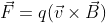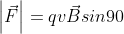=qvB

The path of the particle will be circular

Q5: Define 1 Ampere current in the international unit system.

One Ampere current is the constant current maintained between two straight parallel conductors of infinite length at 1 metre apart and kept in the vacuum of negligible circular cross-section, would produce between these two conductors a force equal to 2 x 10-7 N/m of length.

Q6: What will be the direction of the magnetic field if a proton is moving vertically upwards and the magnetic force acting on it is in a horizontal plane towards the north?

Answer: The direction is calculated using Fleming’s left-hand rule. The direction will be along the west.

Q7: A charged particle does uniform motion in a uniform magnetic field then how would be the path of the particle?

The path of the particle will be linear.

Q8: On any circular coil a constant voltage battery is connected at the diameter ends. What would be the magnetic field at the centre of the coil?

The magnetic field at the centre of the coil will be zero.

Q9: In a current-carrying coil of radius R and having N turns is opened and made into a straight long wire. Then the magnetic field at a distance R would be how many times of the value of the centre of the coil?

The magnetic field at the centre

B0= μ0NI/2R

B = μ0I/2πR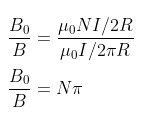Q10: What will be the distance between two points of inflations of a Helmholtz coil?

It is equal to the radius of the coil.

Q11: Write the mathematical form of Ampere’s Circuit Law.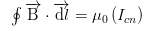Q12: In a long tube of copper having an internal radius R, I current is flowing. Calculate the magnetic field inside the tube.

The magnetic field inside the tube is zero.

Q13: Why are magnetic poles of a moving coil galvanometer made of concave shape?

In order to produce a radial magnetic field magnetic poles are made in a concave shape.

Q14: How is the current sensitivity of a galvanometer increased?

The sensitivity of a galvanometer can be increased by taking a soft iron core or by increasing the number of turns in the coil.

Q15: What will be the position of the magnetic field and the coil of a galvanometer when it is in equilibrium position?

The plane of the coil and magnetic field will be perpendicular.

Q16: Why is cyclotron not used to accelerate light charged particles?

When the energy of the light charged particle is increased there is an increase in the mass of the particle due to relativistic effect.

Q17: Which device will you use to produce a uniform magnetic field?

The device used is Helmholtz’s coil.

Q18: How does the period of revolution of a charged particle depend on the speed and radius of a circular path inside “Dee” of the cyclotron?

The time period will remain constant.

Q19: Write the expression for the high resistance used to convert a galvanometer into

a voltmeter.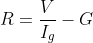Ig is the current required for full-scale deflection

G is the resistance of the galvanometer

V is the range of the voltmeter

Q1: Write down the conclusion from Oersted’s experiment.

1. A magnetic field is produced around a current-carrying conductor.
2. When the magnitude of the electric field is increased then the magnetic field is also increased.
3. The strength of the magnetic field depends on the distance from the current carrying wire. The magnetic field is stronger near the conductor and decreases as the distance from the current conducting wire increases.
4. If the current is in a south-north direction, the north pole of the magnetic field is deflected to the west. If the current is in the north-south direction, the north pole of the magnetic field is deflected to the east. We can conclude that with a change in direction of current, there will be a change in the direction of the magnetic field.
5. The direction of the magnetic field will be always opposite above and below the conductor wire.

Q2: Define Biot-Savart’s law in vector form.

The vector form of Biot-Savart’s law is given by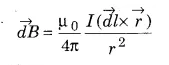Q3: Explain the two laws to find the direction of the magnetic field produced by electric current.

The direction of the magnetic field can be found using the following law

(i) Snow rule: According to snow rule, if the electric current is flowing from south to north in a conducting wire, the needle in the magnetic compass deflects in the west direction.

(ii) Right-Hand Thumb Rule: According to this rule, if the current carrying wire is held in the right hand so that the thumb shows the direction of the current then the curved fingers show the direction of the magnetic field.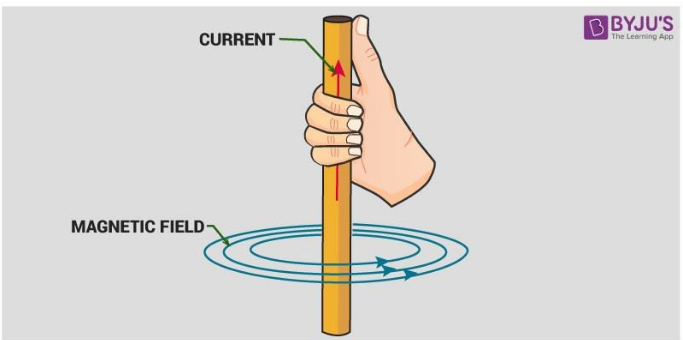Q4: A charge enters a uniform magnetic field at an angle (0くθく90°). What will be the path of the charge? Also, find its pitch.

The particle enters at an angle θ. The velocity of the particle can be resolved into two components. Vcosθ along the magnetic field and Vsinθ perpendicular to the magnetic field.

The path will be straight due to Vcosθ and circular due to Vsinθ. Therefore, the resultant path will be helical.

The radius of the helical path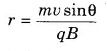The time period of the circular motion is given by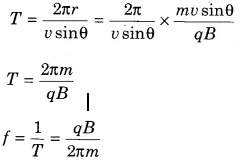The frequency value is the inverse of Time period

Pitch of the helical path

The distance travelled along the axis of the helical path in one time period is called the pitch of the path

Pitch=VcosθxT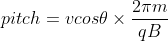Q5: Find the relation between the magnetic field at R/2 on-axis, and magnetic field at the centre of the coil. Here R is the radius of the coil.

The magnetic field due to the circular coil is given by the equation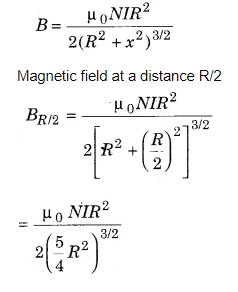Magnetic field at the centre is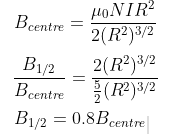Q6: Show how a small current loop behaves like a bar magnet?

When there is current flow through a loop, the loop behaves like a bar magnet. The face at which the current is anticlockwise is called the north pole and the face at which current is clockwise is called the south pole.

Q7: What is the circulation of the magnetic field? Please explain

Circulation of current means if the field B is directed along the tangent to every point on the perimeter L of a closed curve and its magnitude is constant along the curve. Then BL=μ0I

Q8: What is the difference in the behaviour of a solenoid and bar magnet?

1. In solenoid the magnetic field lines are parallel and in the bar magnet they are curved.
2. Outside solenoid the magnetic field is nearly zero and outside the bar magnet, it is along its length.

Q9: Find the force per unit length on two parallel current-carrying conductors.

Any two current-carrying conductors when placed near each other, will exert a magnetic force on each other.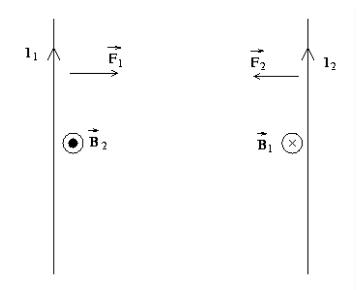Consider the system shown in the figure above. Here, we have two parallel Current carrying conductors, separated by a distance ‘d’, such that one of the conductors is carrying a current I1 and the other is carrying I2. We can say that conductor 2 experiences the same magnetic field at every point along its length due to conductor 1. The direction of magnetic force is indicated in the figure and is found using the right-hand thumb rule. The direction of the magnetic field, as we can see, is downwards due to the first conductor.

From the Ampere’s circuital law, the magnitude of the field due to the first conductor can be given by,

B1 = μ0I1/2πd

The force on a segment of length L of the conductor 1 due to the conductor 2 can be given as,

F2 =I2B1lsin900 = I2B1l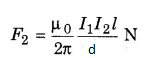Similarly, the force on a segment of length L of the conductor 2 due to conductor 1 can be given as,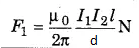We see that conductor 1 experiences the same force due to the conductor 2 but the direction is opposite. Thus,

F1 = F2

Thus, we can say force per unit length on two parallel current-carrying conductors is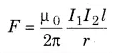Q10: Show the period of half revolution of a positive charge in “Dee” of a cyclotron does not depend on the radius of the circular path.

For cyclotron

mv2/r = qvB

Where m is the mass of the charged particle, v is the velocity of the charged particle and B is the magnetic field.

r=mv/qB

Time period T= 2πr/v

Substituting the value of r in the equation of time period we get

T = 2πm/qB

The time taken to complete half-circle is

T/2 = πm/qB

Q11: Explain the principle of the cyclotron.

The Principle of Cyclotron is that when a particle is moving normal to the magnetic field it will experience a Lorentz force because of which the particle moves in a circular path. This is explained in detail below

1. The dees are placed opposite to each other with a narrow gap between them. The particles can move in the gap. The particles are put in the centre of the gap.
2. The dees are kept between the two poles of an electromagnet.
3. The magnetic field causes the particles to take a circular path due to the Lorentz force
4. A very high alternating voltage is applied between the dees. The voltage creates an alternating field which accelerates the particles.
5. The frequency of the voltage is adjusted such that the particles make one circle during one cycle of voltage.The frequency must be set to the particle’s cyclotron frequency.

Q12: What is sensitivity and figure of merit of a galvanometer? How are they related to each other?

Sensitivity of galvanometer

Sensitivity is the ratio of change in deflection of the galvanometer to the change in current/voltage in the coil.

Figure of merit

Figure of merit is the ratio of the full-scale deflection current and the number of graduations on the scale of the instrument. It gives the value of the current required to produce a unit deflection in the galvanometer.

Figure of merit is inversely proportional to the current sensitivity of a galvanometer.

Q13: Find the expression for the resistance connected in parallel to convert a galvanometer to an ammeter.

For the conversion of the galvanometer into the ammeter, shunt resistance is required. The shunt resistance is usually made from copper wire and its resistance is much lesser than the galvanometer.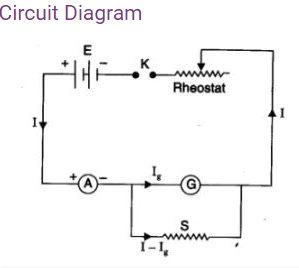In the given figure I is the main current Ig is the current passing through the galvanometer for maximum deflection. Then the current passing through the shunt resistance is Is= I – Ig.

The shunt and galvanometer are connected in parallel and hence the potential difference will be the same

IgG=IsS

G is the resistance of the galvanometer and S is the resistance of the shunt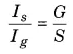Let us add 1 on both sides of the equation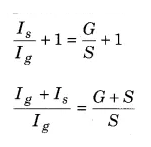The total current I=Ig+Is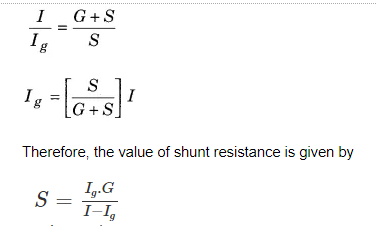Q14: A rectangular current loop EFGH is placed in a uniform magnetic field, as shown in the figure

1. What will be the direction of torque on loop?
2. When torque will be (i) maximum (ii) zero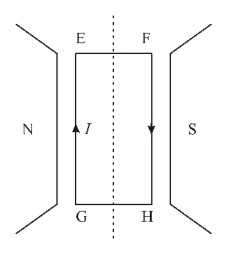1. According to the right-hand rule, the direction of the torque is perpendicular to the plane of paper inwards.
2. 1. When Ө=90 degrees

τmax=NIABsin90

τmax= NIAB

2. When Ө=0

τmin =NIABsin0

τmin=0

Q1: What is a galvanometer? Describe the construction and working of a galvanometer using a labeled diagram.

A moving coil galvanometer is an instrument which is used to measure electric currents. It is a sensitive electromagnetic device, which can measure low currents even of the order of a few microamperes.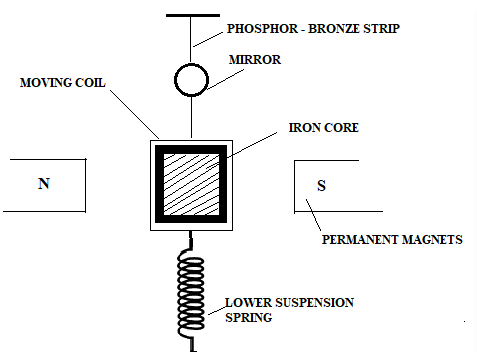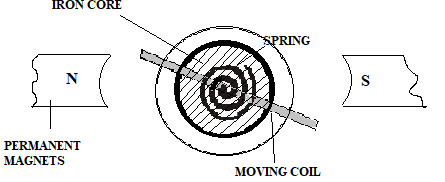Construction

The moving coil galvanometer has a rectangular coil that has many turns and it is usually made of thinly insulated or fine copper wire that is wounded on a metallic frame. The coil is free to rotate about a fixed axis. A phosphor-bronze strip is connected to a movable torsion head. This is used to suspend the coil in a uniform magnetic field.

A cylindrical soft iron core is symmetrically positioned inside the coil to improve the strength of the magnetic field and to make the field radial. The lower part of the coil is attached to a phosphor-bronze spring having a small number of turns. The other end of the spring is connected to binding screws.

The spring is used to produce a counter torque which balances the magnetic torque and hence helps in producing a steady angular deflection. A plane mirror which is attached to the suspension wire, along with a lamp and scale arrangement is used to measure the deflection of the coil. Zero-point of the scale is at the centre.

Working

Let a current I flow through the rectangular coil of n number of turns and a cross-sectional area A. When this coil is placed in a uniform radial magnetic field B, the coil experiences a torque τ.

Let us first consider a single turn ABCD of the rectangular coil having a length l and breadth b. This is suspended in a magnetic field of strength B such that the plane of the coil is parallel to the magnetic field. Since the sides AB and DC are parallel to the direction of the magnetic field, they do not experience any effective force due to the magnetic field. The sides AD and BC being perpendicular to the direction of field experience an effective force F given by F = BIl

Using Fleming’s left-hand rule we can determine that the forces on AD and BC are in opposite directions to each other. When equal and opposite forces F called a couple acts on the coil, it produces a torque. This torque causes the coil to deflect.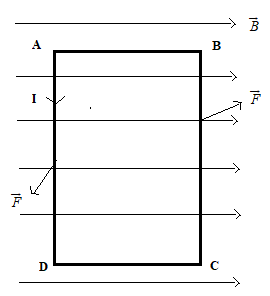We know that torque τ = force x perpendicular distance between the forces

τ = F × b

Substituting the value of F we already know,

Torque τ acting on single-loop ABCD of the coil = BIl × b

Where lx b is the area A of the coil,

Hence, the torque acting on n turns of the coil is given by

τ = nIAB

The magnetic torque thus produced causes the coil to rotate, and the phosphor bronze strip twists. In turn, the spring S attached to the coil produces a counter torque or restoring torque kθ which results in a steady angular deflection.

Under equilibrium condition:

kθ = nIAB

Here k is called the torsional constant of the spring (restoring couple per unit twist). The deflection or twist θ is measured as the value indicated on a scale by a pointer which is connected to the suspension wire.

θ= ( nAB / k)I

Therefore, θ ∝ I

The quantity nAB / k is a constant for a given galvanometer. Hence, it is understood that the deflection that occurs in the galvanometer is directly proportional to the current that flows through it.